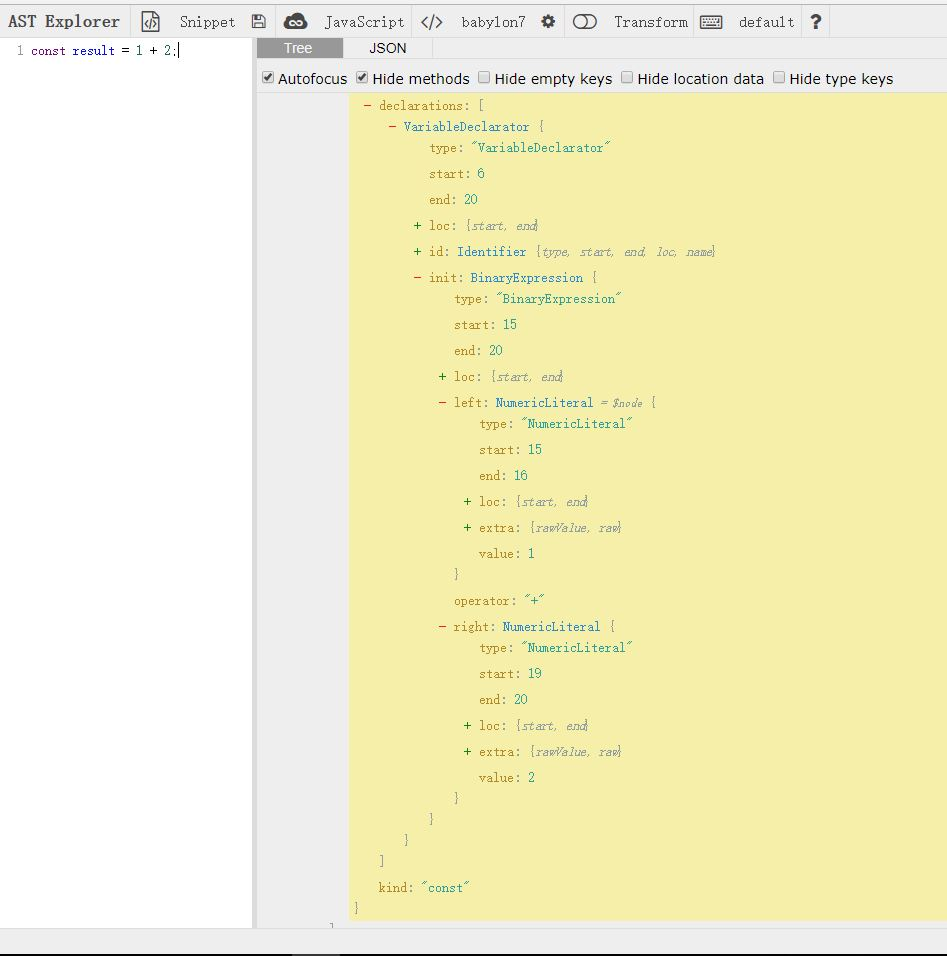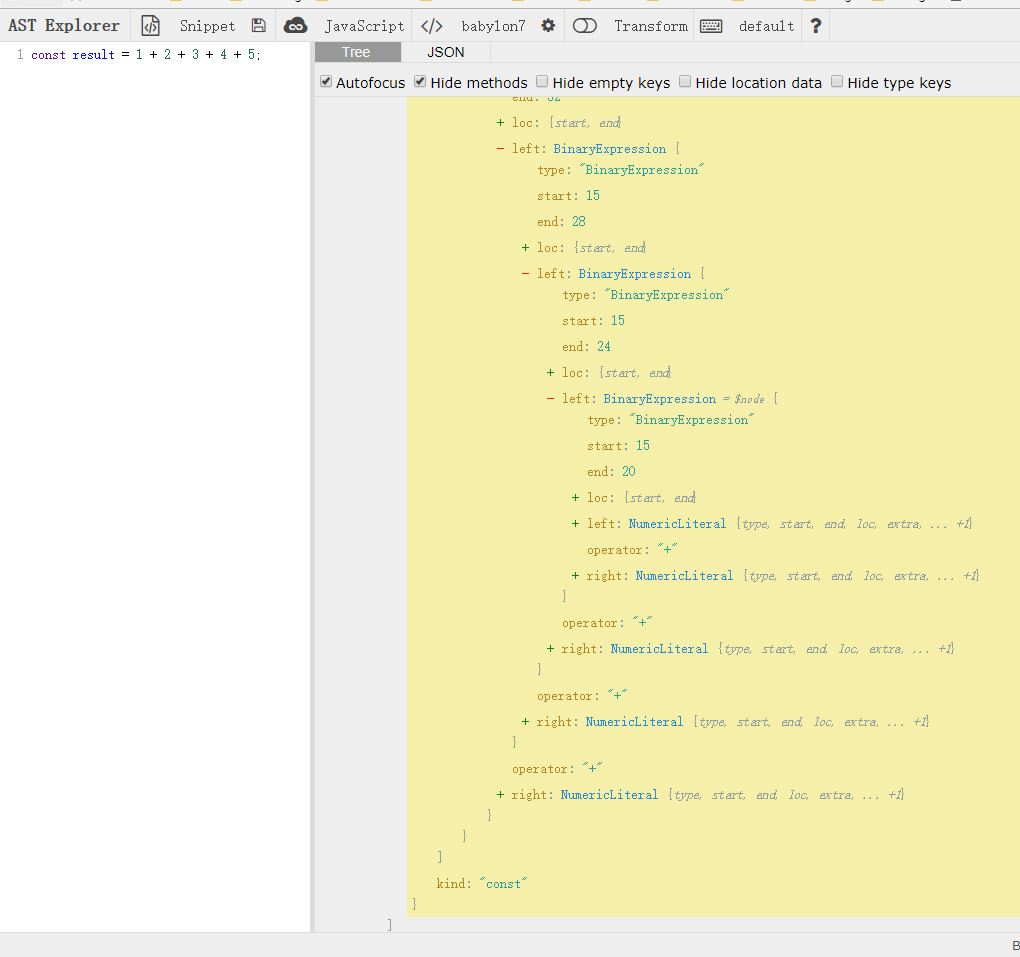1. 你了解过Babel吗？

1. 写过Babel插件吗

1. 如果让你写一个插件，你能写的出来吗?

### 预览

Before:

``````const result = 1 + 2 + 3 + 4 + 5;
``````

After:

``````const result = 15;
``````

``````setTimeout(function(){
// do something
}, 1000 * 2) // 插件要做的事，就是把 1000 * 2 替换成 2000
``````

### 开工

``````module.export = function(babel){
return {
visitor:{
}
}
}
``````

visitor是对各类型的AST节点做处理的地方，那么我们怎么知道Babel生成了的AST有哪些节点呢？``````var babel = require('babel-core');
var t = require('babel-types');

const visitor = {
BinaryExpression(path) {
const node = path.node;
let result;
// 判断表达式两边，是否都是数字
if (t.isNumericLiteral(node.left) && t.isNumericLiteral(node.right)) {
// 根据不同的操作符作运算
switch (node.operator) {
case "+":
result = node.left.value + node.right.value;
break
case "-":
result = node.left.value - node.right.value;
break;
case "*":
result =  node.left.value * node.right.value;
break;
case "/":
result =  node.left.value / node.right.value;
break;
case "**":
let i = node.right.value;
while (--i) {
result = result || node.left.value;
result =  result * node.left.value;
}
break;
default:
}
}

// 如果上面的运算有结果的话
if (result !== undefined) {
// 把表达式节点替换成number字面量
path.replaceWith(t.numericLiteral(result));
}
}
};

module.exports = function (babel) {
return {
visitor
};
}
``````

``````const babel = require("babel-core");

const result = babel.transform("const result = 1 + 2;",{
plugins:[
require("./index")
]
});

console.log(result.code); // const result = 3;
````````````表达式( 表达式( 表达式( 表达式(1 + 2) + 3) + 4) + 5)
``````

``````    // 判断表达式两边，是否都是数字
if (t.isNumericLiteral(node.left) && t.isNumericLiteral(node.right)) {}
``````

``````表达式( 表达式( 表达式(3 + 3) + 4) + 5)
``````

``````表达式( 表达式(6 + 4) + 5)

15
``````
``````    // 如果上面的运算有结果的话
if (result !== undefined) {
// 把表达式节点替换成number字面量
path.replaceWith(t.numericLiteral(result));

let parentPath = path.parentPath;

// 向上遍历父级节点
parentPath && visitor.BinaryExpression.call(this, parentPath);
}
``````

`const result = 100 + 10 - 50` >>> `const result = 60;`

`const result = (100 / 2) + 50` >>> `const result = 100;`

`const result = (((100 / 2) + 50 * 2) / 50) ** 2` >>> `const result = 9;`

### 完结

51 回复AST 的用处挺广的，除了 Babel，还会用在 WebPack，CodeMod，ESLint 等地方。 我写过后面 2 个：

• CodeMod（ jscodeshift）用于编写代码自动升级工具
• ESLint 用于自定义静态代码检查，如最近刚写了个：

• 你是怎么在项目做工程化的

• 你项目的架构是怎么样

• 如何编写一个公共库

• 你了解过Javascript哪些前沿的技术

Prettier、Prepack、(区块链，机器学习…), 刚讲完前面那两个，估计觉得太low，没让我讲下去了2333

@axetroy 来广州的话可以试试我这边

@atian25 egg-bin check这个，以前我就有这样的困惑，既然"约定大于规范", 都是约定好的。

@atian25 广州哪里可以投递呢

@atian25 感觉扫描的约定是不错，但是对 TS 的支持不太好，因为通过 load 加载会类型丢失，还要自己加类型声明，我觉得应该 TS 优先会更好，要是有什么机制可以把这些声明自动加到 tsserver 里面就好了。直接加入 ts-node/register 就可以支持导入 ts ，但是因为我看 loadconfig 里面都是把后缀给写死了的，所以 config 根本加载不进去。

@axetroy 你在 「Egg 非官方自助交流群」 么？在的话加下我微信。

egg-bin check这个，以前我就有这样的困惑，既然"约定大于规范", 都是约定好的。 那么开发者怎么知道你约定了哪些，每次都得去翻阅文档，不然很容易写错，这不像Typescript有IDE的提示。

ts + egg 这块的 RFC 我正在编写中，回头欢迎加入一起讨论：

• 不需要手写 d.ts，egg loader 导致的类型丢失，可以通过 egg-bin 这层自动生成 d.ts 解决
• ts-node 那个我也看了，直接加载 ts 文件的话要修改 loader，这块还需要 @popomore 那边加进来讨论，现在是直接 tsc 编译即可。

@MiYogurt 装饰器这块，是一个语法糖类型的，目前不是我思考 ts-egg 的关键，主要是为了解决 egg loader 导致的类型丢失问题和一些开发辅助。

@atian25 不在哦， 哪里申请

@atian25 加了

@xtx1130 老哥，问一下，parse5生成的ast树，有什么好的方法遍历吗？有无类似babel-traverse的这个方法暴露出来？

@liaoyinglong dom.childNodes.find+dom.nodeName.match 你自己查一下api

@xtx1130 3q 这个看过了，是类似于jq这种直接操作dom的，不太想要这种方法。

@liaoyinglong find功能不就是类似于traverse 的visitor吗？你是有什么功能用find不能实现吗？还有这也不是直接操作dom啊。。。只不过api比较像而已啊,你仔细读读文档

`` .......``

@atian25 看你github上最近关注了他，没想到这么快就下手了😂

@flyguolai 最近老板给了不少 HC，有兴趣的欢迎勾搭

@atian25 二维码过期了T-T 求新的

@atian25 vscode 主题是什么？

@atian25 T-T 超过100人扫不进去了，微信号 sfyr111 求拉一波^^

``````// 单纯的计算的话，在退出节点时处理就行了
visitor: {
BinaryExpression: {
// 退出节点
exit(path) {
const node = path.node;
let result;
// 判断表达式两边，是否都是数字
if (t.isNumericLiteral(node.left) && t.isNumericLiteral(node.right)) {
// 根据不同的操作符作运算
switch (node.operator) {
case "+":
result = node.left.value + node.right.value;
break;
case "-":
result = node.left.value - node.right.value;
break;
case "*":
result = node.left.value * node.right.value;
break;
case "/":
result = node.left.value / node.right.value;
break;
case "**":
let i = node.right.value;
while (--i) {
result = result || node.left.value;
result = result * node.left.value;
}
break;
default:
}
}

// 如果上面的运算有结果的话
if (result !== undefined) {
// 把表达式节点替换成number字面量
path.replaceWith(t.numericLiteral(result));
}
}
}
}
``````